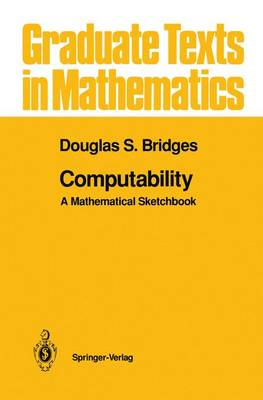•# Computability: A Mathematical Sketchbook - Graduate Texts in Mathematics 146 (Hardback)

(author)
£56.99
Hardback 180 Pages / Published: 14/01/1994
• We can order this

Usually dispatched within 3 weeks

Aimed at mathematicians and computer scientists who will only be exposed to one course in this area, Computability: A Mathematical Sketchbook provides a brief but rigorous introduction to the abstract theory of computation, sometimes also referred to as recursion theory. It develops major themes in computability theory, such as Rice's theorem and the recursion theorem, and provides a systematic account of Blum's complexity theory as well as an introduction to the theory of computable real numbers and functions. The book is intended as a university text, but it may also be used for self-study; appropriate exercises and solutions are included.

Publisher: Springer-Verlag New York Inc.
ISBN: 9780387941745
Number of pages: 180
Weight: 1000 g
Dimensions: 235 x 155 x 12 mm
Edition: 1994 ed.